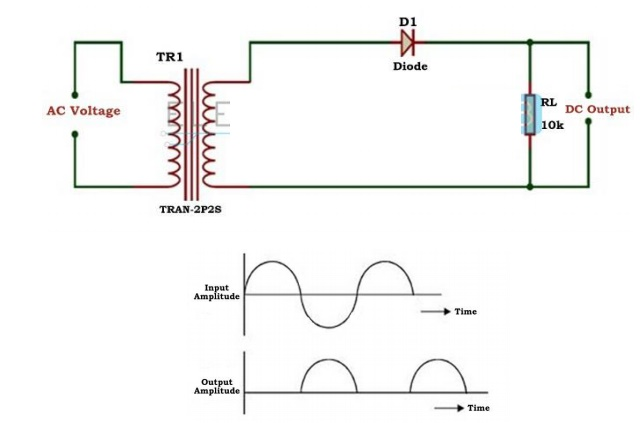Home | | Electronic Devices and Circuits | Half Wave Rectifier: Characteristics and Working

# Half Wave Rectifier: Characteristics and Working

The half wave rectifier is a type of rectifier that rectifies only half cycle of the waveform. This describes the half wave rectifier circuit working.

RECTIFIERS

Rectifiers are classified according to the period of conduction.

They are

Ø        Half Wave Rectifier

Ø        Full Wave Rectifier

## 1. Half Wave Rectifier:

The half wave rectifier is a type of rectifier that rectifies only half cycle of the waveform. This describes the half wave rectifier circuit working. The half rectifier consist a step down transformer, a diode connected to the transformer and a load resistance connected to the cathode end of the diode. The circuit diagram of half wave transformer is shown below:The main supply voltage is given to the transformer which will increase or decrease the voltage and give to the diode. In most of the cases we will decrease the supply voltage by using the step down transformer here also the output of the step down transformer will be in AC. This decreased AC voltage is given to the diode which is connected serial to the secondary winding of the transformer, diode is electronic component which will allow only the forward bias current and will not allow the reverse bias current. From the diode we will get the pulsating DC and give to the load resistance RL.

2. Working of Half Wave Rectifier:

The input given to the rectifier will have both positive and negative cycles. The half rectifier will allow only the positive half cycles and omit the negative half cycles. So first we will see how half wave rectifier works in the positive half cycles.### Ø        Positive Half Cycle:

·                    In the positive half cycles when the input AC power is given to the primary winding of the step down transformer, we will get the decreased voltage at the secondary winding which is given to the diode.

·                    The diode will allow current flowing in clock wise direction from anode to cathode in the forward bias (diode conduction will take place in forward bias) which will generate only the positive half cycle of the AC.

·                    The diode will eliminate the variations in the supply and give the pulsating DC voltage to the load resistance RL. We can get the pulsating DC at the Load resistance.

### Ø        Negative Half Cycle:

·                    In the negative half cycle the current will flow in the anti-clockwise direction and the diode will go in to the reverse bias. In the reverse bias the diode will not conduct so, no current in flown from anode to cathode, and we cannot get any power at the load resistance.

·                    Only small amount of reverse current is flown from the diode but this current is almost negligible. And voltage across the load resistance is also zero.

## 3. Characteristics of Half Wave Rectifier:

There are some characteristics to the half wave rectifier they are

Ø        Efficiency: The efficiency is defined as the ratio of input AC to the output DC.

Efficiency, Ƞ = P dc / Pac

DC power delivered to the load, Pdc = I2dc RL = ( Imax/pi ) 2 RL

AC power input to the transformer, Pac = Power dissipated in junction of diode + Power dissipated in load resistance RL

= I2rms RF + I2rms RL = {I2MAX/4}[RF + RL]

Rectification Efficiency, Ƞ = Pdc / Pac = {4/ 2}[RL/ (RF + RL)] = 0.406/{1+ RF/RL } If RF is neglected, the efficiency of half wave rectifier is 40.6%.

Ø        Ripple factor: It is defined as the amount of AC content in the output DC. It nothing but amount of AC noise in the output DC. Less the ripple factor, performance of the rectifier is more. The ripple factor of half wave rectifier is about 1.21 (full wave rectifier has about 0.48). It can be calculated as follows:

The  effective  value  of  the  load  current  I is  given  as  sum  of  the  rms  values  of

Harmonic currents I1, I2, I3, I4 and  DC current Idc.

I2 =I2dc+I2 1+I22+I24  = I2    dc +I2ac

Ripple factor, is given as γ = I ac / Idc = (I2 – I2dc) / Idc = {( I rms / Idc2)-1} = Kf2 – 1)

Where Kf is the form factor of the input voltage. Form factor is given as

Kf = Irms /Iavg = (Imax/2)/ (Imax/pi) = pi/2 = 1.57

So, ripple factor, γ = (1.572 – 1) = 1.21

Ø        Peak Inverse Voltage: It is defined as the maximum voltage that a diode can with stand in reverse bias. During the reverse bias as the diode do not conduct total voltage drops across the diode. Thus peak inverse voltage is equal to the input voltage Vs.

Ø        Transformer Utilization Factor (TUF): The TUF is defined as the ratio of DC power is delivered to the load and the AC rating of the transformer secondary. Half wave rectifier has around 0.287 and full wave rectifier has around 0.693.

Half wave rectifier is mainly used in the low power circuits. It has very low performance when it is compared with the other rectifiers.

Study Material, Lecturing Notes, Assignment, Reference, Wiki description explanation, brief detail
Electronic Devices and Circuits : PN Junction Devices : Half Wave Rectifier: Characteristics and Working |